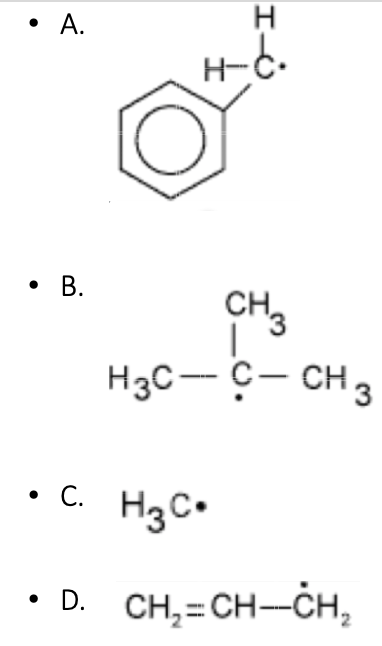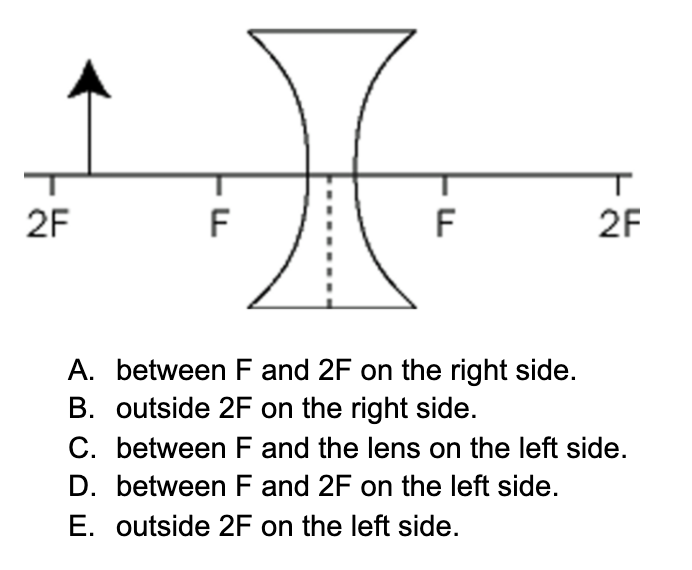# Free OAT Practice Questions

If you don’t have time for a full OAT practice test, and you need just a few sample OAT questions to see where you stand, you’ve come to the right place.

## OAT Practice Questions

Which of the following illustrates the principle of induction in invertebrates?

• A. In an embryo, the presence of a notochord beneath the ectoderm results in the formation of a neural tube.
• B. A neuron synapses with another neuron via a neurotransmitter.
• C. Eye muscles constrict in response to light.
• D. Secretion of TSH stimulates the secretion of thyroxine.
• E. The maternal parent induces spontaneous expression of recessive genes.

C6H12O6 + O2 CO2 + H2O

This process is completed

• A. in the cytoplasm.
• B. in the area of the cell membrane.
• C. in the nucleus.
• D. in the mitochondria.
• E. in the area around the ribosomes.

Cells that are involved in active transport, such as cells of the intestinal epithelium, utilize large quantities of ATP. In such cells there are

• A. high levels of adenylate cyclase activity.
• B. many polyribosomes.
• C. many mitochondria.
• D. high levels of DNA synthesis.
• E. many lysosomes.

In an emergency, an individual with type AB antigen on his red blood cells

• A. may receive a transfusion of type O blood.
• B. may receive a transfusion of type A blood.
• C. may receive a transfusion of type B blood.
• D. All of the above
• E. None of the above

The least stable free radical isWhich one of the following reactions will be accompanied by an increase in entropy?

• A. Na(s) + H₂O(l)  → NaOH(aq) + H₂(g)
• B. I₂(g) I₂(s)
• C. H₂SO₄(aq) + Ba(OH)₂(aq) →  BaSO₄(s) + H₂O(l)
• D. H₂(g) + 1/2 O₂ (g) → H₂O(l)
• E. None of the above.

The heat of combustion of gaseous ammonia, NH₃(g), is 81 kcal/mole. How much heat is released in the reaction of 34 grams of ammonia with excess oxygen?

• A. 40.5 kcal
• B. 60.3 kcal
• C. 75.8 kcal
• D. 81 kcal
• E. 162 kcal

Which of the following electron configurations represents the element that would form the most acidic anhydride?

• A. 1s²2s²2p⁶3s¹
• B. 1s²2s²2p⁶3s²
• C. 1s²2s²2p⁶3s²3p¹
• D. 1s²2s²2p⁶3s²3p²
• E. 1s²2s²2p⁶3s²3p³

A roulette wheel consists of 38 slots. 36 of these are numbered 1 through 36 and colored red or black so that there are nine red even-numbered slots, nine black odd-numbered slots, etc. These slots occur with equal probability. The two slots marked 0 or 00 are each three times as likely to occur as any one of the other 36. What is the probability that a red even number, a red 23, or a 00 will occur on one roll of the wheel?

• A. 2/7
• B. 5/18
• C. 13/38
• D. 13/42
• E. 3/10

If 1/5 of those in a high school are freshmen, and 40% of the freshmen are women, and 3/5 of those in the other grades are women, how many men are enrolled in the high school, given that there are 600 students altogether?

• A. 240
• B. 264
• C. 300
• D. 336
• E. 360

In the first 12 games of the season, the forward on a soccer team scored 20 goals. How many goals must he average in each of the remaining 6 games of the season in order to average 2.0 goals per game overall?

• A. 1 ½
• B. 2 ⅓
• C. 2 ½
• D. 2 ⅔
• E. 20

Susan has \$10 with which to buy apples. Apples are priced at 6 for \$0.89, or \$0.20 each. How much change will Susan receive if she buys as many as she can?

• A. \$1.10
• B. \$0.89
• C. \$0.21
• D. \$0.10
• E. \$0.01

When a spring is compressed to its minimum length and not permitted to expand

• A. potential energy is at its maximum and kinetic energy is at its minimum.
• B. kinetic energy is at its maximum and potential energy is at its minimum.
• C. the sum of the potential and kinetic energies is zero.
• D. potential energy and kinetic energy are at their maximum.
• E. potential energy and kinetic energy are at their minimum.

A 5-N force and a 10-N force act on the same point of an object. The 5-N force acts due west while the 10-N force acts 60° north of east. The resultant force is

• A. 5 N east
• B. 5√3 N north
• C. 5 N northwest
• D. 5√3 N northeast
• E. 5 N south

An object is placed near a biconcave lens as shown below. The image will formWhich of the following statements concerning the photoelectric effect is FALSE?

• A. The kinetic energy of the emitted electrons is equal to the energy of the light waves.
• B. As the intensity of the light increases, the number of electrons emitted per unit time should increase.
• C. If the frequency of the light wave increases and the intensity remains constant, the kinetic energy of the emitted electrons increases.
• D. If light of extremely low frequency is incident upon a photosensitive metallic surface, an electron will not be emitted regardless of how long the light is incident upon that surface.
• E. One can measure the kinetic energy of emitted electrons by measuring the voltage of the electric field necessary to stop the motion of the electrons.

Complete the quiz above to get your score and see the correct answers. For a sample question every day, try the OAT question of the day.